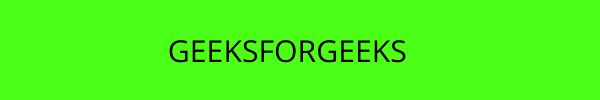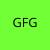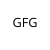• Difficulty Level : Expert
• Last Updated : 23 Sep, 2021

We can easily add moving platforms in our arcade game by following the below steps:

• Create 2 new variables for sprite and velocity of moving platform.
```self.moving_vel = 3
self.moving_platform = None```
```self.moving_platform = arcade.Sprite("Moving.png",1)
self.moving_platform.center_x = 500
self.moving_platform.center_y = 500```
• Update the y-coordinate of the platform.
`self.moving_platform.center_y -= self.moving_vel`
• Change the direction of the platform if the platform crosses the boundary.
```if self.moving_platform.center_y < 100 or self.moving_platform.center_y>600:
self.moving_vel *= -1```

### Sprites Used:For this, we are going to create a MainGame() class. Inside this class first, we are going to initialize some variables for player’s velocity, platform velocity, camera, player sprite, physics engine, and our scene. After that, we will create 6 functions inside this class.

• on_draw(): Inside this function, we will use our camera, and then we will draw our scene.
• setup(): In this function, we will initialize our camera and scene object then we will load our player and platform’s sprites. After that, we will call the  PhysicsEnginePlatformer() function.
• on_update(): In this function, we will update the x coordinate of the player’s sprite by adding the value of the velocity variable and we also update the y coordinate of our moving platform. After that, we will call our camera_move() function.
• on_key_press(): In this function, we will change the value of the vel_x  variable according to the keyboard key that is pressed.
• on_key_release(): In this function, we will change the value of the vel_x variable according to the keyboard key that is released.
• camera_move(): In this function, we will change the position of the camera according to the current position of the player.

Below is the implementation:

## Python3

 `# Importing arcade module``import` `arcade`` ` `# Creating MainGame class       ``class` `MainGame(arcade.Window):``    ``def` `__init__(``self``):``        ``super``().__init__(``600``, ``600``, title``=``"Player Movement"``)`` ` `        ``# Initializing a variable to store``        ``# the velocity of the player``        ``self``.vel_x ``=` `0`` ` `        ``# Creating variable for Camera``        ``self``.camera ``=` `None`` ` `        ``# Creating variable for moving velocity``        ``self``.moving_vel ``=` `3`` ` `        ``# Creating variable for Platform``        ``self``.moving_platform ``=` `None``         ` `        ``# Creating scene object``        ``self``.scene ``=` `None`` ` `        ``# Creating variable to store player sprite``        ``self``.player ``=` `None`` ` `        ``# Creating variable for our game engine``        ``self``.physics_engine ``=` `None`` ` `    ``# Creating on_draw() function to draw on the screen``    ``def` `on_draw(``self``):``        ``arcade.start_render()`` ` `        ``# Using the camera``        ``self``.camera.use()``         ` `        ``# Drawing our scene``        ``self``.scene.draw()`` ` `    ``def` `setup(``self``):``         ``# Initialize Scene object``        ``self``.scene ``=` `arcade.Scene()``         ` `        ``# Using Camera() function``        ``self``.camera``=` `arcade.Camera(``600``,``600``)`` ` `        ``# Creating different sprite lists``        ``self``.scene.add_sprite_list(``"Player"``)``        ``self``.scene.add_sprite_list(``"Platforms"``, use_spatial_hash``=``True``)`` ` `        ``# Adding player sprite``        ``self``.player_sprite ``=` `arcade.Sprite(``"Player.png"``, ``1``)`` ` `        ``# Adding coordinates for the center of the sprite``        ``self``.player_sprite.center_x ``=` `64``        ``self``.player_sprite.center_y ``=` `600`` ` `        ``#Adding Sprite in our scene``        ``self``.scene.add_sprite(``"Player"``, ``self``.player_sprite)`` ` `        ``#Adding moving Platform sprite``        ``self``.moving_platform ``=` `arcade.Sprite(``"Moving.png"``,``1``)`` ` `        ``# Adding starting coordinates``        ``self``.moving_platform.center_x ``=` `500``        ``self``.moving_platform.center_y ``=` `500`` ` `        ``# Adding to Platforms sprite group ``        ``self``.scene.add_sprite(``"Platforms"``,  ``self``.moving_platform)`` ` `        ``# Adding platform sprite according to level``        ``platform ``=` `arcade.Sprite(f``"Platform.png"``, ``1``)``         ` `        ``# Adding coordinates for the center of the platform``        ``platform.center_x ``=` `300``        ``platform.center_y ``=` `32``        ``self``.scene.add_sprite(``"Platforms"``, platform)`` ` `        ``# Creating Physics engine``        ``self``.physics_engine ``=` `arcade.PhysicsEnginePlatformer(``            ``self``.player_sprite,``          ``self``.scene.get_sprite_list(``"Platforms"``),``          ``0.5``        ``)`` ` ` ` `    ``# Creating on_update function to``    ``# update the x coordinate``    ``def` `on_update(``self``,delta_time):`` ` `        ``# Changing x coordinate of player``        ``self``.player_sprite.center_x ``+``=` `self``.vel_x ``*` `delta_time``         ` `        ``# Updating the physics engine to move the player``        ``self``.physics_engine.update()`` ` `        ``# Adding moving velocity in moving platform``        ``self``.moving_platform.center_y ``-``=` `self``.moving_vel`` ` `        ``# Changing the direction ``        ``if` `self``.moving_platform.center_y < ``100` `or``        ``self``.moving_platform.center_y>``600``:``            ``self``.moving_vel ``*``=` `-``1`` ` `        ``# Calling the camera_move function``        ``self``.camera_move()`` ` `    ``# Creating function to change the velocity``    ``# when button is pressed``    ``def` `on_key_press(``self``, symbol,modifier):`` ` `        ``# Checking the button pressed``        ``# and changing the value of velocity``        ``if` `symbol ``=``=` `arcade.key.LEFT:``            ``self``.vel_x ``=` `-``300``        ``elif` `symbol ``=``=` `arcade.key.RIGHT:``            ``self``.vel_x ``=` `300``        ``elif` `symbol ``=``=` `arcade.key.UP:``            ``if` `self``.physics_engine.can_jump():``                ``self``.player_sprite.change_y ``=` `15`` ` `    ``# Creating function to change the velocity``    ``# when button is released``    ``def` `on_key_release(``self``, symbol, modifier):`` ` `        ``# Checking the button released``        ``# and changing the value of velocity``        ``if` `symbol ``=``=` `arcade.key.LEFT:``            ``self``.vel_x ``=` `0``        ``elif` `symbol ``=``=` `arcade.key.RIGHT:``            ``self``.vel_x ``=` `0`` ` `    ``def` `camera_move(``self``):``        ``# Getting the x coordinate for the center of camera``        ``screen_x ``=` `self``.player_sprite.center_x ``-``        ``(``self``.camera.viewport_width ``/` `2``)``         ` `        ``# Getting the y coordinate for the center of camera``        ``screen_y ``=` `self``.player_sprite.center_y ``-``        ``(``self``.camera.viewport_height ``/` `2``)``         ` `        ``# Moving the camera``        ``self``.camera.move_to([screen_x, screen_y])``         ` `# Calling MainGame class       ``game ``=` `MainGame()``game.setup()``arcade.run()`

Output:My Personal Notes arrow_drop_up